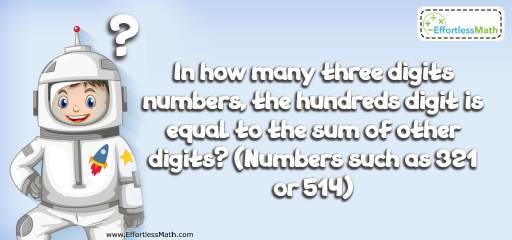# Number Properties Puzzle -Critical Thinking 5

Let's try a little mental exercise with this critical thinking puzzle related to Number Properties. All you need is your brain and a sense of logic.## Challenge:

In how many three digits numbers, the hundreds digit is equal to the sum of other digits? (Numbers such as 321 or 514)

### The Absolute Best Book to challenge your Smart Student!

One way to answer to this question is considering all three digits numbers and find those that follow the rules. Another way is finding the pattern.
The hundreds digit equals to other digits. Let’s start with 1 as hundreds digit. 1 equals to sum of 1 and 0. We can find two such numbers; 101 and 110.
Now, let’s consider 2. Number 2 equals to the sum of 1 and 1 or 2 and 0. We can find one number for the first and two numbers for the second pair. 211, 202 and 220.
Number 3 equals to the sum of 3 and 0 or 2 and 1. Therefore, the numbers are 303, 330, 312, 321
Number 4 equals to the sum of 4 and 0, or 3 and 1, or 2 and 2.
The numbers are: 404, 440, 413, 431, 422.
With the same method, we can find the following numbers for 5 as hundreds digit:
505, 550, 514, 541, 532, 523.
For number 6 as hundred digits:
606, 660, 615, 651, 624, 642, 633
Now, use the pattern:
For 1 as hundreds place, there are 2 numbers.
For 2 as hundreds place, there are 3 numbers.
For 3 as hundreds place, there are 4 numbers.
For 4 as hundreds place, there are 5 numbers.
….
Therefore, the total three digits numbers in which the hundreds digit is equal to the sum of other digits equals to:
2 + 3 + 4 + 5 + 6 + 7 + 8 + 9 + 10 = 54

### What people say about "Number Properties Puzzle -Critical Thinking 5 - Effortless Math: We Help Students Learn to LOVE Mathematics"?

No one replied yet.

X
30% OFF

Limited time only!

Save Over 30%

SAVE $5 It was$16.99 now it is \$11.99